Education

# 25 Engaging Online Interactive Math Games for Every Grade Level

Looking for fun ways to get kids to do some math practice? Try these online interactive math games! They’re perfect for at-home enrichment or homework assignments. We also love playing them on an interactive projector because you can enlarge the display, project the game on a wall or table, and play as a class. Use your finger or the interactive pen to control the gameplay from the board. Alternatively, you can have students play the games on their individual devices in the classroom, periodically sharing their work or questions on your projector for you to give feedback.

It’s important to spark an interest in math at a young age and build confidence in skills quickly. Fun interactive math games are a great way to do it.

## Ten-Frame Mania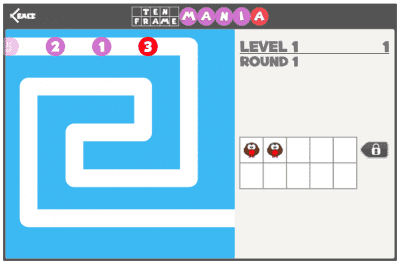What students practice: Counting and ten-frames

As the numbers come onto the screen, students click the boxes in the ten-frame to match the number. It disappears and they start over with the next number.

## Puzzle Pics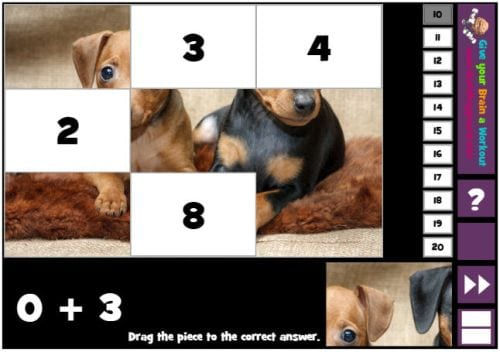What students practice: Basic addition and subtraction

Click and drag the puzzle pieces to answer the math questions, revealing a cool picture at the end. There are multiple versions for different skills and levels, and you can change the target sums too.

Play it: Puzzle Pics at Math Playground

## Bar Graphing With Eggs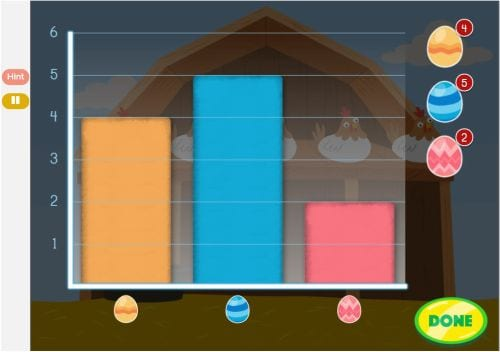What students practice: Sorting, graphing

First, move the frying pan to catch the colored eggs as they fall from the chickens. Then, sort the eggs by pattern. Finally, use the eggs to create a basic bar graph.

## Math Baseball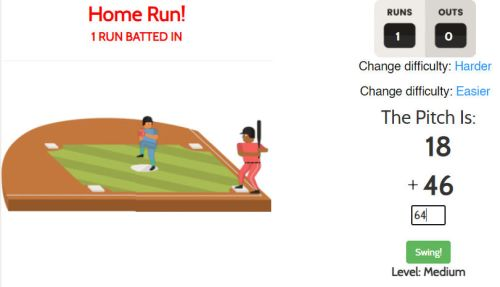What students practice: Arithmetic skills

It’s a simple concept: Students solve addition, subtraction, multiplication, and division equations, then take a swing. You can change the difficulty from easy to “super brain,” so this is a game that grows with kids.

Play it: Math Baseball at Funbrain

## Number Bonds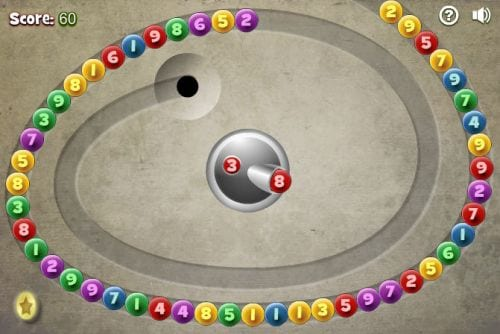Choose a target sum between 10 and 20. Then, aim and shoot the center number ball at one of the balls circling the track to make the target sum. Repeat until all the balls are gone.

Where to find it: Number Bonds at Math Playground

## Candy Cashier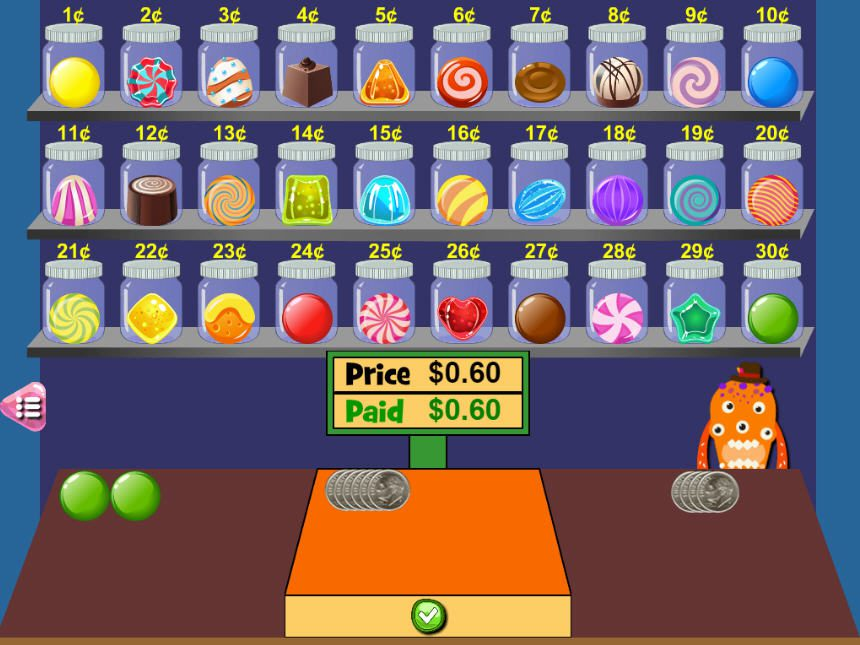What students practice: Adding up money and making change

Welcome to the monster candy shop! As each monster comes in to make a purchase, add up the cost. Then, use their money to make the appropriate payment.

Play it: Candy Cashier at Math Playground

## Estimation Contraption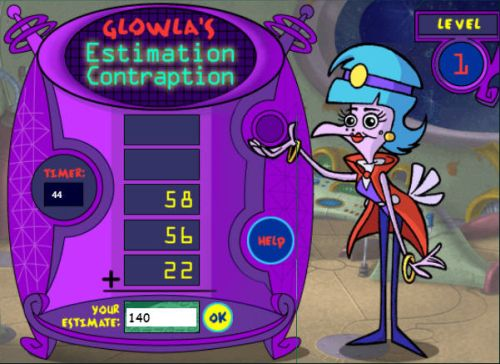What students practice: Estimation and addition

Estimation seems simple, but it can give some kids real trouble. Play this simple game to get practice rounding and adding numbers.

Play it: Estimation Contraption

## Coin Weighing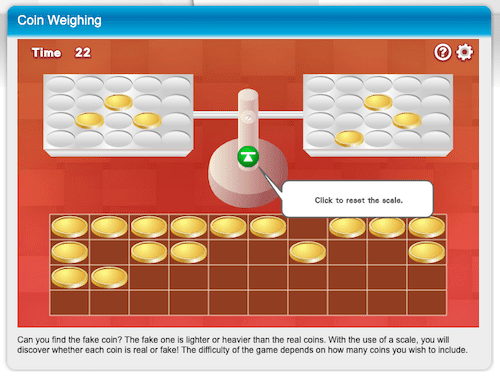What students practice: Comparison, weights

Students have four chances to weigh coins to determine which one is fake. In addition to practicing weights, kids have to think carefully to solve the problem.

Play it: Coin Weighing at Math Game Time

## Math Pac-Man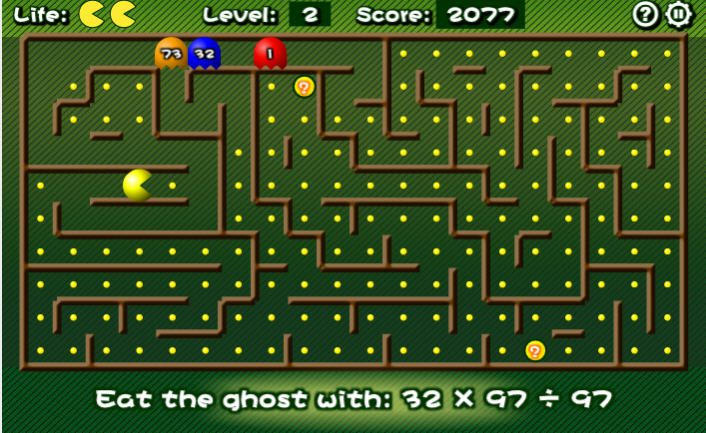What students practice: Arithmetic equations

Classic arcade game meets math! You’ll have to think fast to solve the equation and eat the right ghost. Try this one in pairs with one student solving and the other moving Pac-Man as fast as they can.

Play it: Math Pac-Man at Math Game Time

## Canoe Puppies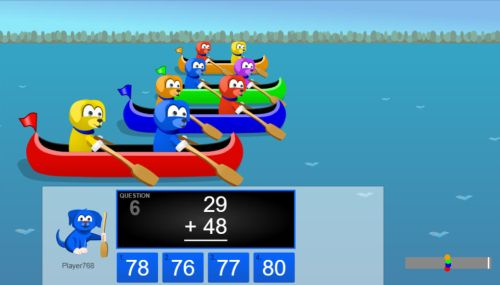Can your puppy-filled canoe beat the others to the finish line? Solve the equations quickly to paddle your way to victory!

Play it: Canoe Puppies at Math Playground

## Kangaroo Hop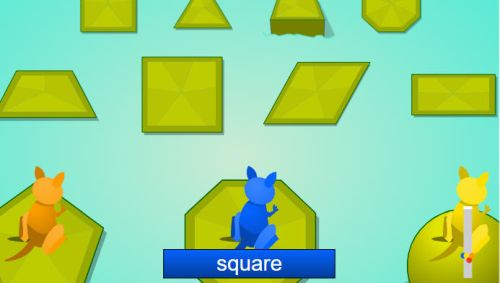What kids learn: Geometric shapes

Hop from shape to shape faster than your opponents. Students need to know both 2D and 3D shapes to master this one.

Play it: Kangaroo Hop at Math Game Time

As math becomes more challenging, it can also become more difficult to have student engagement. Entertaining math games help students sharpen their skills without feeling like a bore.

## Orbit Integers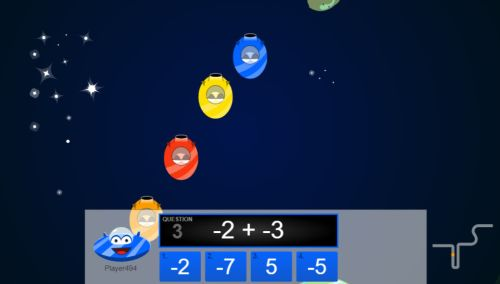What students learn: Adding and subtracting positive and negative integers

Can you win the space race? Students need to solve the equations faster than everyone else in the competition to soar into first.

## Math in Music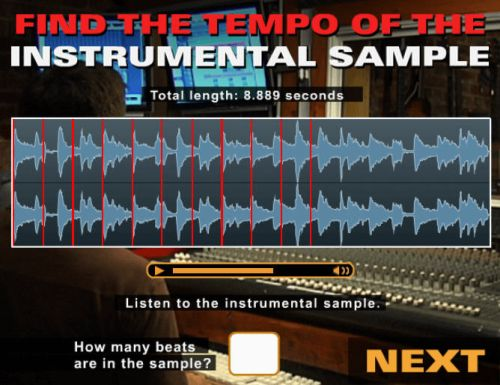What students practice: Real-world algebra skills

Students always ask how they’ll use math in real life, and this game gives an example. The challenge is to adjust the music tracks so they align. First, though, they have to figure out how many beats per minute in each. How will they solve the problem?

Play it: Math in Music at Get the Math

## Puppy Chase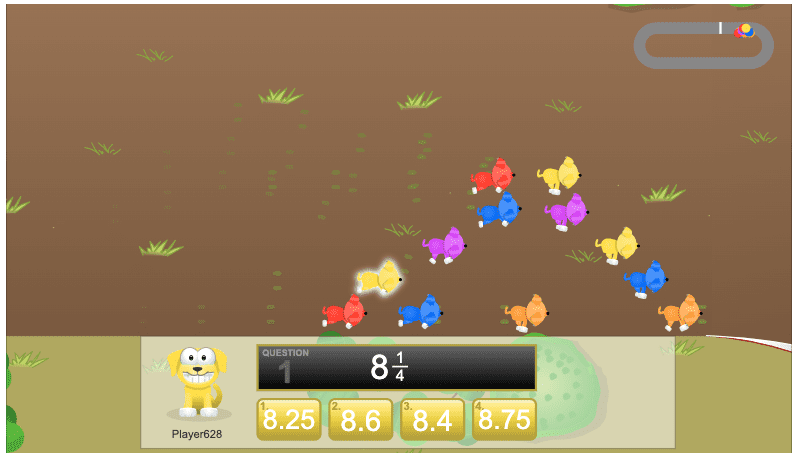What students practice: Decimals and equivalent fractions

Click the equivalent decimal from the fraction given. The faster you find the answers, the more you move ahead to win the race!

Play it: Puppy Chase at Math Playground

## Find the Quark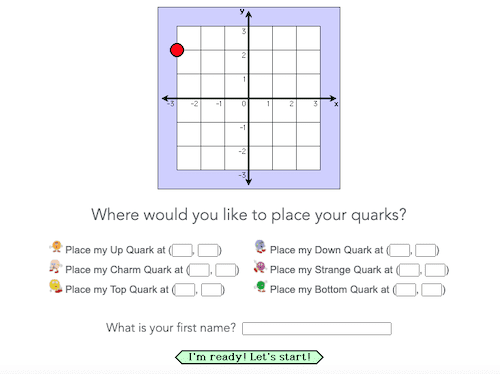What students practice: Coordinate graphing

If kids have played Battleship, they’ll recognize this game. This time, they’re looking for the Quarks hidden somewhere on the board.

Where to find it: Find the Quark at JLab

## High-Stakes Heist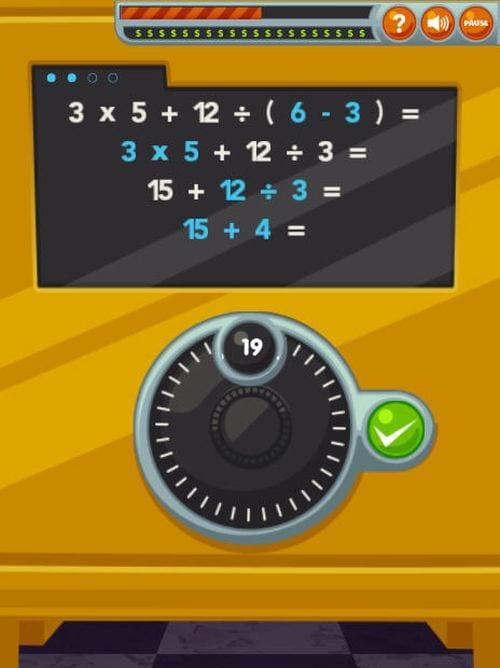What students practice: Order of operations

Crack the safe by solving an equation, one step at a time, using the correct order of operations. If you make a mistake, you can go back to the previous step to fix it.

Play it: High-Stakes Heist at ABCYa

## Algebraic Reasoning Sweet Shop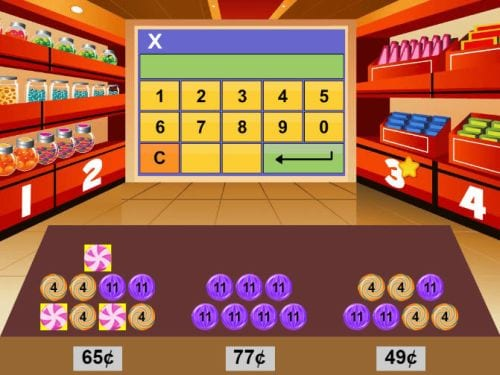What students practice: Mental math, writing equations

Using the stacks of various candies that add up to certain amounts, determine how much each individual candy is worth. This can be done as mental math or by writing out and solving equations.

Where to find it: Algebraic Reasoning Sweet Shop at Math Playground

## Boat Coordinates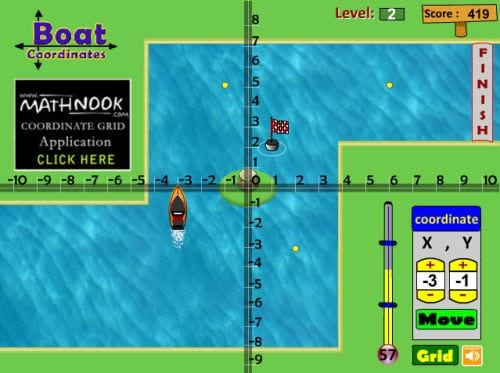What students practice: Coordinate graphing

Enter the coordinates to send the boat on its way to the finish line. Stop along the way to pick up coins but be sure to avoid obstacles. You have a limited amount of time, so think fast! This game can be customized with single-quadrant or four-quadrant boards.

Where to find it: Boat Coordinates at Math Nook

Higher-level math doesn’t have to be all work and no play. Online math games for high school students are harder to find, but these games give students the chance to practice their skills in unique ways.

## Exponents Jeopardy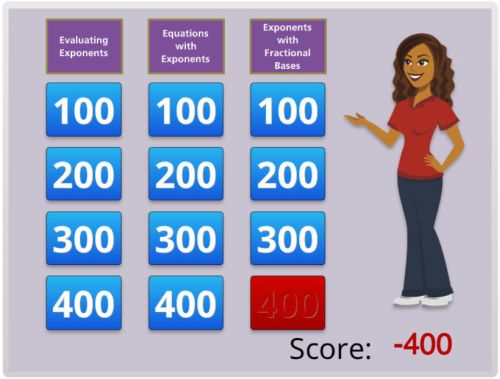What students practice: Exponents

You know the drill: Pick a category, solve the equation, earn the points. Be careful though! If you’re wrong, you can lose all the points you’ve earned.

## Wrecks Factor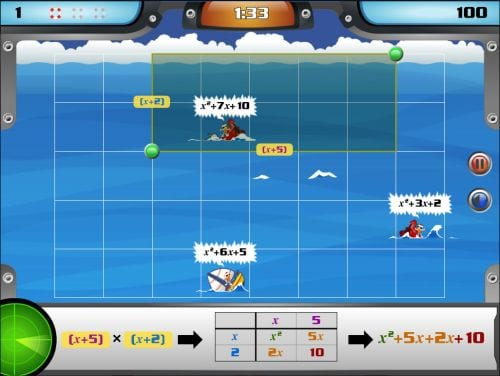What students practice: Factoring quadratic equations

Ships sail onto the board and wreck, displaying a quadratic equation. Factor the equation, then click and drag the correct answer to the points on the grid that surround the boat. More boats continue to arrive, and if one sinks before you solve the equation, you lose a life.

Where to find it: Wrecks Factor at Mangahigh

## Math in Fashion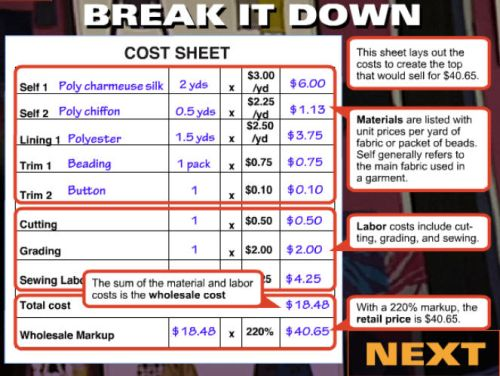What students practice: Decimals, multiplication, problem-solving

See how math plays a role in fashion design as you try to alter the design of a blouse to meet the target price. This is a fun game to play together in groups so you can see how others would make the changes.

Play it: Math in Fashion at Get the Math

## Transformation Golf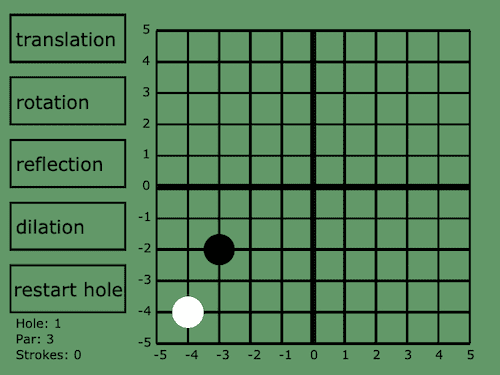What students practice: Translation and transformation in a coordinate plane

Pick a transformation and then a factor choice of that transformation to get the golf ball into the hole. Includes translation, rotation, reflection, and dilation.

## Sortify: Angles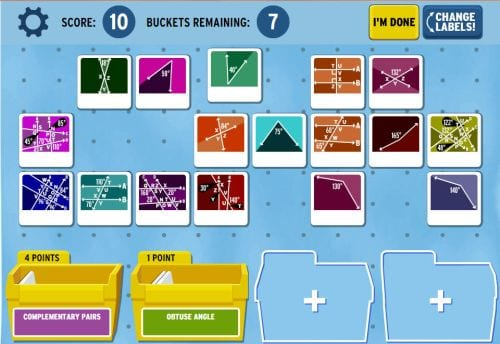What students practice: Types of angles, basic geometry

Sort the cards by dragging them into bins and placing the correct label on them. Some cards need to be linked together, like those that form complementary or supplementary angles. Submit the bins to be checked and earn points for correct answers.

Play it: Brainpop

## Math in Videogames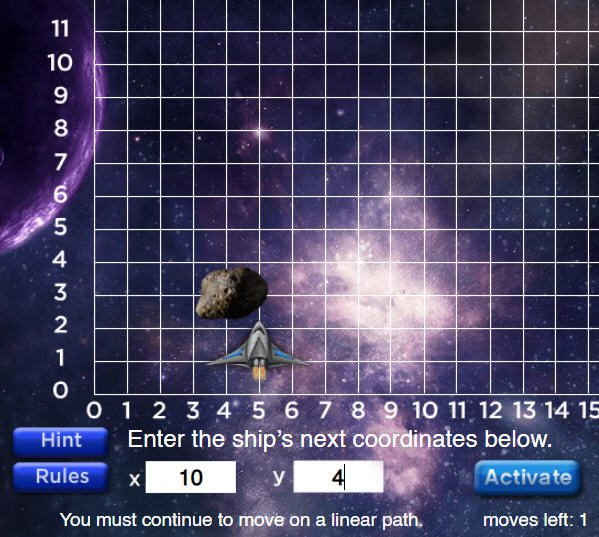What students practice: Graphing on a coordinate plane

Students practice graphing linear paths on a coordinate plane with this game, and keep the spaceship from crashing into the asteroid.

## Geogebra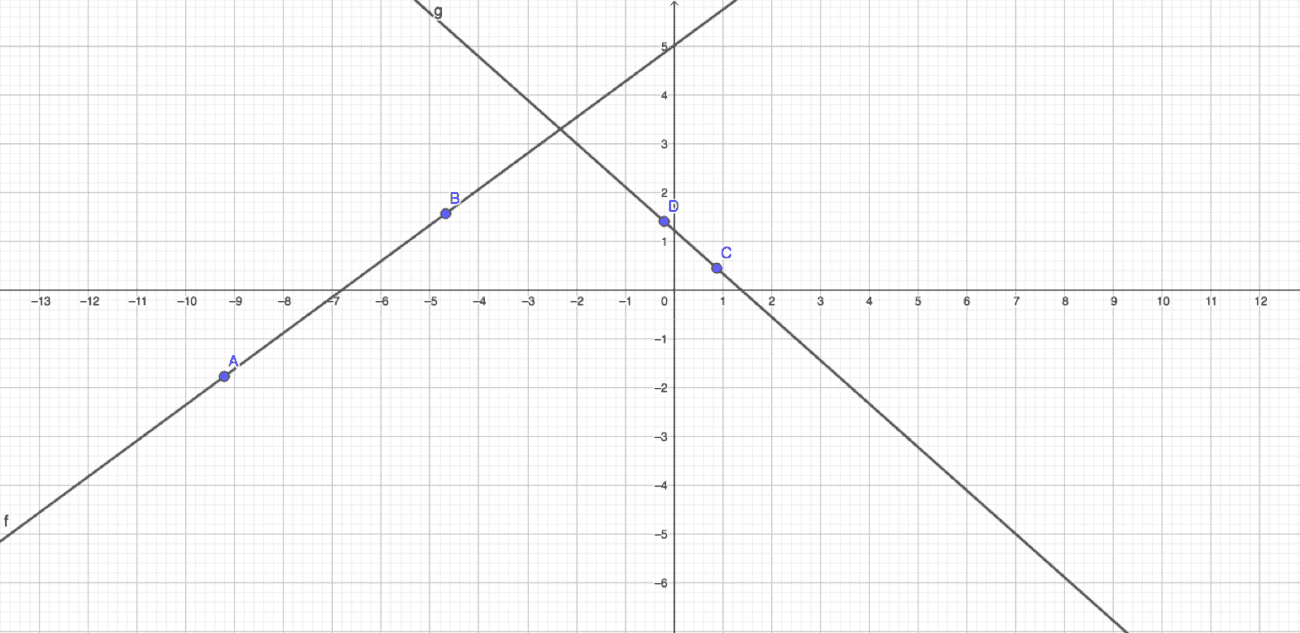What students practice: Geometry

While not a game per se, we love the interactive site Geogebra for creating graphs, 3D shapes, and more. It’s a perfect way to add an interactive touch to your geometry lessons.

Where to find it: Geogebra